# Conditional branching in JavaPhoto by Jon Tyson on Unsplash

Conditional branching is a logical part of the program. Based on the specific condition we can tell to program which way the program should go.

`if <it's cold outside> then I will get my jacketelse I'm good with T-shirt `

The above example shows a simple version of conditional branching in plain language. `<it's cold outside>` this is a logical condition and it can be true or false. If it’s true then we will perform some action and if it’s false we can perform another action.

The logical condition can be stored in `boolean` type variable and can be used as part of the conditional branching statements(if-esle, ternary operator).

`// class and main method is not displayedboolean b = true;if (b) {   System.out.println("Java is great!");}else {   System.out.println("Python is great!");}`

in above the snippet we can see the simple if-else statement.

• The output of this program is `Java is great!`
• The if statement always takes boolean datatype as its condition, between the parenthesis. If the condition true if’s body will be executed and if it’s false else’s body will be executed.
• This code will always print `Java is great!` because we hardcoded the value of our boolean as true. Usually, we get boolean from some conditions (comparison, event, etc.).

# Comparison Operators

Let’s talk about how we can get a logical boolean. One way to get it is by using comparison operators.

• `==` equal to operator. We can use this operator to compare primitive datatypes on equality. For objects, it will compare if two references are pointing to the same object or not(we will talk more in the future).
• `!=` not equal to operator. Exactly same as equal, but works in reverse.
• `>` greater than operator. To compare if one number greater than another.
• `>=` greater than or equal to operator. To compare if one number greater or equal to another number.
• `<` less than
• `<=` less than or equal to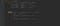in Main.java

Using `==` to compare numbers on equality.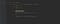in Main.java file

The output from the above program is `Samsung`. The not equal operator is the exact opposite of equal to operator. If numbers are not equal it will return true and if they are equal it will return false.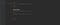in Main.java

The greater than and less than operators are very straightforward. if you want to also check greater/less or equal you need add `=` after the operator. Like `>=` and `<=`

# Logical Operators

Write a method that will accept one number argument. If number within a range 10–20 inclusive print `Java` otherwise, print `Python`.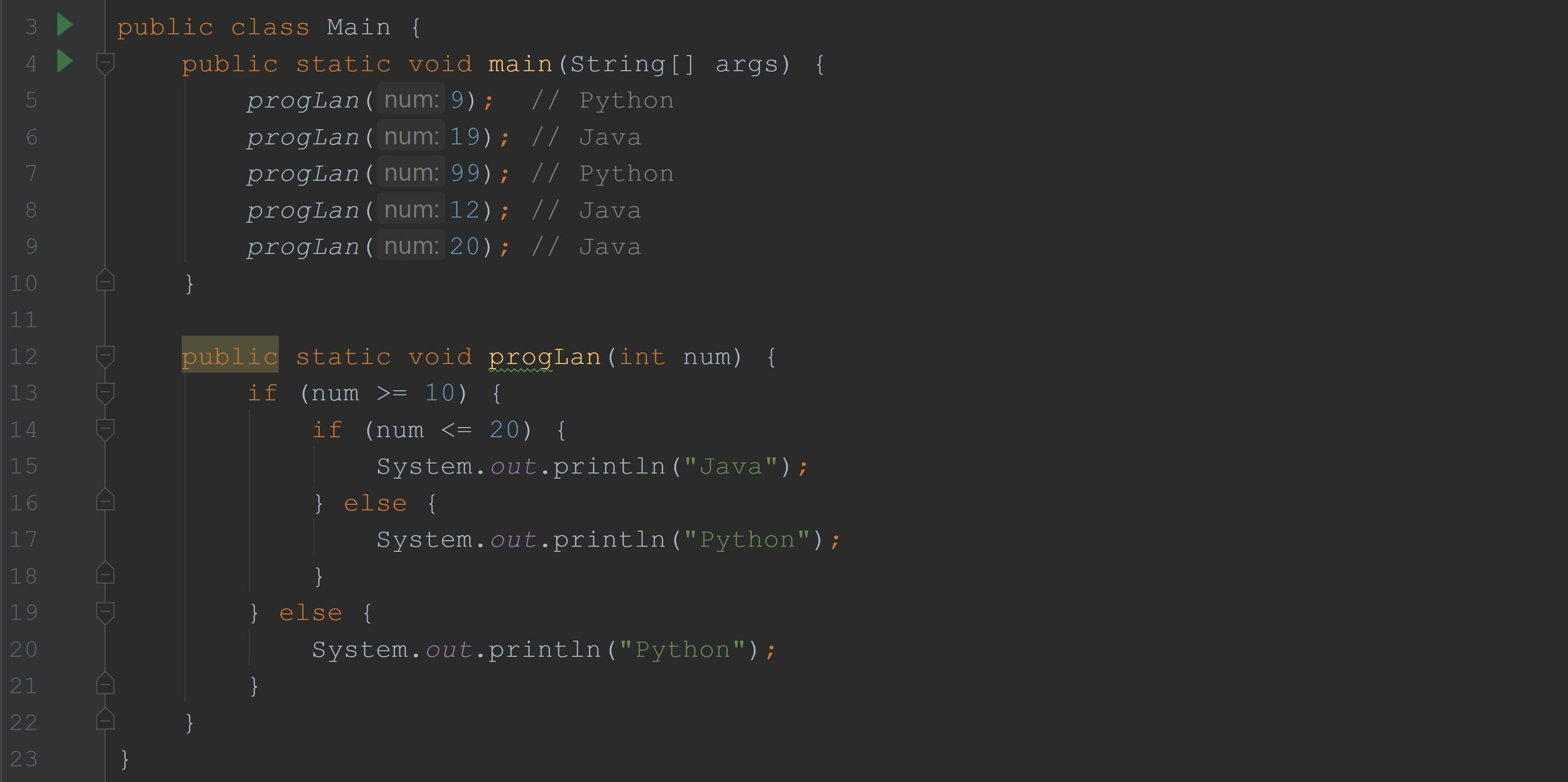in Main.java

Even though, above code works well for our requirement, it’s not the best way of achieving it. For this simple condition, we used two if-else statements. Now let’s see code using a logical operator.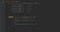in Main.java

Here we used the logical AND(&&) operator. The logical operators are super useful to connect boolean conditions into one. They help us to make our conditions clean and short. When using AND operator all conditions should be true then only the whole condition will be true.

• `&&` logical AND operator
• `||` logical OR operator
• `!` logical NOT operator

Write a mathod that will accept one number as an argument.
Assume numbers 7, 13, 99 are lucky numbers. If the number is lucky, print `Jackpot!` otherwise, print `Not today!`.

With the OR operator if at least one part true then the whole condition is true.

Logical NOT operator will reverse value of boolean.

`boolean b = true;boolean c = !b;boolean d = !false;System.out.println(b); // trueSystem.out.println(c); // falseSystem.out.println(d); // trueint money = 99;if (!(money >= 100)) {    System.out.println("It's not enough");}`

We can use logical NOT just in front of the boolean condition and it will reverse its value. Notice how we use parentheses. Parentheses clarify the meaning of a boolean expression. Also, they are useful when many conditions are used together to clarify and separate.

# Multibranch if-else

Write a method that will accept one double number and will print a message based on this number:
- if the number within a range of 13.5–15.5 inclusive, print `normal pressure`
- if the number is less than 13.5 exclusives, print `low pressure`
- otherwise, print `high pressure`

`public void printPressureMsg(double pressure) {   if (pressure >= 13.5 && pressure <= 15.5) {      System.out.println("normal pressure");   }else if (pressure < 13.5) {      System.out.println("low pressure");   }else {      System.out.println("high pressure");   }}`

As you can see here we can connect if statements into one statement. Only one of them will be executed and if all do not match else statement will be executed. Else part is optional. It’s always better to have multibranch if-else than having multiple if statements separately.

write a method that accepts one number and will print a message based on
- if the number is even print `Fizz`
- if the number is odd print `Buzz`
- if the number is odd and divisible by 5 evenly print `FizzBuzz`

`public void fizzBuzz(int num) {   if (num % 2 == 0) {      System.out.println("Fizz");   } else if (num % 2 == 1) {      System.out.println("Buzz");    } else if (num % 2 == 1 && num % 5 == 0) {      System.out.println("FizzBuzz");   }}`

It seems like a correct method but there a defect in the code. For most of the number it will work fine but for example, for `15` it will print `Buzz` but it should print `FizzBuzz`.

Order of the if/else if is very important. We discussed that only one statement will be executed even though a few of them can true. So in our case `num % 2 == 1` is true and `num % 2 == 1 && num % 5 == 0` is also true. And correct one is the second one but the first one is first by order so it will always catch this condition and get executed.

`public void fizzBuzz(int num) {   if (num % 2 == 0) {      System.out.println("Fizz");   } else if (num % 2 == 1 && num % 5 == 0) {      System.out.println("FizzBuzz");    } else if (num % 2 == 1) {      System.out.println("Buzz");   }}`

This is the correct version.

This article is part of the series of articles to learn Java programming language from Tech Lead Academy.

`1. Introduction to programming 2. OS, File, and File System3. Working with terminal 4. Welcome to Java Programming Language5. Variables and Primitives in Java6. Methods wiht Java7. Conditional branching, comparision and logical operators8. Switch statemet and ternary operator9. Enum10. String and its methods in Java11. Loops in Java12. Class, Object and constructor in Java13. Object Oriented Programming in Java14. Encapsulation in Java15. Inheritance in Java16. Abstraction in Java17. Polymorphism in Java18. Overriding vs Overloading in Java19. OOP Design Principles in Java20. Array in Java21. Data Structures with Java22. Collection framework in Java23. ArrayList in Java24. Set in Java25. Map in Java26. LocalDate in Java27. Exception in Java28. IO in Java29. Design Patterns30. JUnit`

## More from Beknazar

Software Developer, Java Instructor https://www.techleadacademy.io/